# Computing the Libration Points

Equations for the Libration Points

The libration points, also called the equilibrium or Lagrangian points, are solutions to the equations of motion for the spacecraft such that the x, y, and z positions and x, y, and z velocities are all 0. So to compute the location of these points, one must solve the equations of motion under these special conditions. When solving, one finds that there are 5 solutions to these equations:
• Two solutions fall out easily; each forms and equilateral triangle with the earth and the sun in the plane of rotation
• Each of the 3 remaining solutions also lie in the plane of rotation, on the line between the earth and sun

These solutions are shown and labeled below: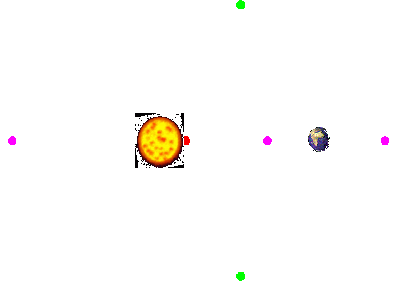To find the location of the 3 libration points lying on the earth-sun axis (L1, L2, and L3), one must solve for the x coordinate of each. This boils down to solving a quintic polynomial equation. It can be proven that each polynomial has one and only one root for mu in the range of 0 to 1.

The equations for L1, L2, and L3 along with the graphs of the corresponding quintic polynomials are shown below for several values of mu:

L1: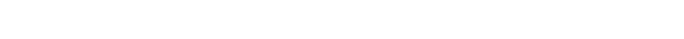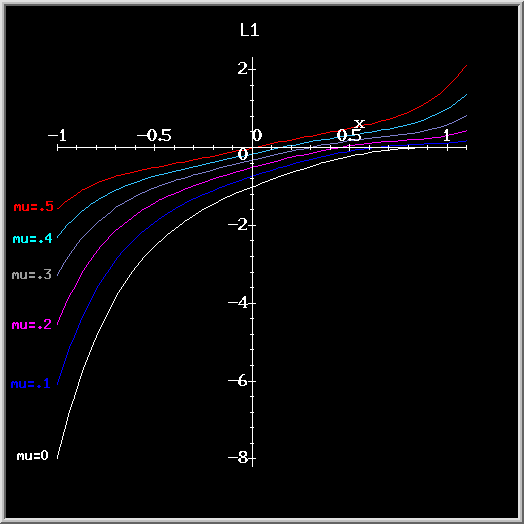L2: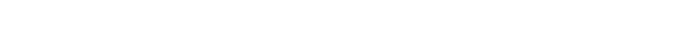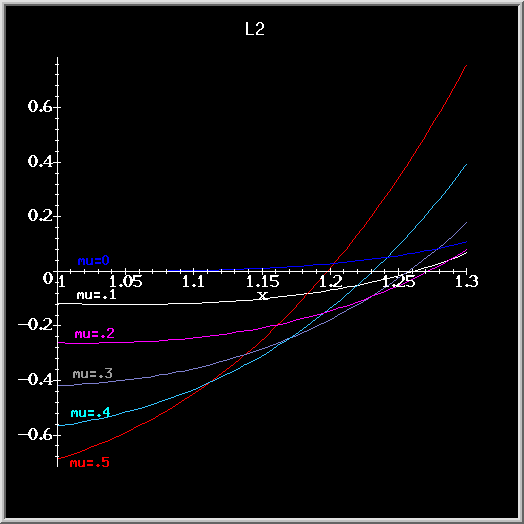L3: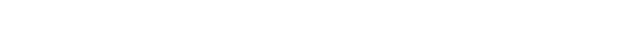Two basic observations can be made by looking at the graphs above:

1. The region of each polynomial curve about the point x=0 is well behaved in the sense that it does not have any inflection points
2. The polynomial zeros for each libration point vary smooothly with the parameter mu

This suggests the method that we use in our project to compute the x coordinate of L1, L2, and L3:

1. Write a function to approximate the zero of each polynomial based on the value of mu by using a curve fit
2. Use Newton's method to find the zero to the desired precision

Once the libration points have been located, one can search for orbits about these points.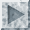How to Begin the Orbit Search